Next: The notion of a grammar Up: Grammars and Parsing Previous: Grammars and Parsing

## An introduction to the notion of a grammar

Informally, a GRAMMAR describes the hierarchical structure of programming language constructs. For instance an if-then-else statement in C has the form
if (expression) statement else statement
Using the variable expr to denote an expression and the variable stmt to denote a statement, the above rule can be reformulated as follows in order to express that an if-then-else statement is a particular statement
stmt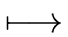if (expr) stmt else stmt
Such formulation will called a PRODUCTION.

Example 1   Another example is that of arithmetic expressions over arbitrary variables (i.e. expression involving variables, the four arithmetic operations and exponentiation) which can be defined by the following productions (or grammatical rules):
 exprexpr binop expr expr(expr) expr-expr exprid binop+ binop- binop* binop/ binop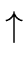where id stands for any variable.

Remark 1   Let I be a finite set of identifiers and let us consider the alphabet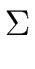=   I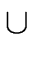{ + , - ,*,/,,(,)}. (1)

Let L be the language consisting of arithmetic expressions over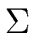. We shall show that L cannot be recognized by FA.

First we observe that every word w = w1 ... w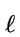of L is a well parenthesized expression that is

 (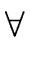i = 1 ...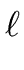- 1)  | w1 ... wi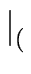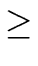| w1 ... wi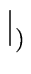and    | w= | w(2)

where | u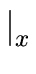denotes the number of occurrences of the letter x in the word u. The proof is easy by induction.

Let us assume that L can be recognized by a DFA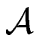= (, S, s0, F,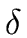) with N states and initial state s0. Let us consider an arithmetic expression a starting with N opening parentheses. There exists N states s1, s2,..., sN such that for every i = 1 ... N - 1 we have

 si+1  =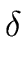(si, x)    with    x = ( (3)

There exists at least two values i1 and i2 of the index i in the range 0 ... N such that si1 = si2. Hence by adding i2 - i1 opening parentheses in front of a we can build another word b recognized by L. However b is clearly not a well parenthesized expression and thus it is not an arithmetic expression.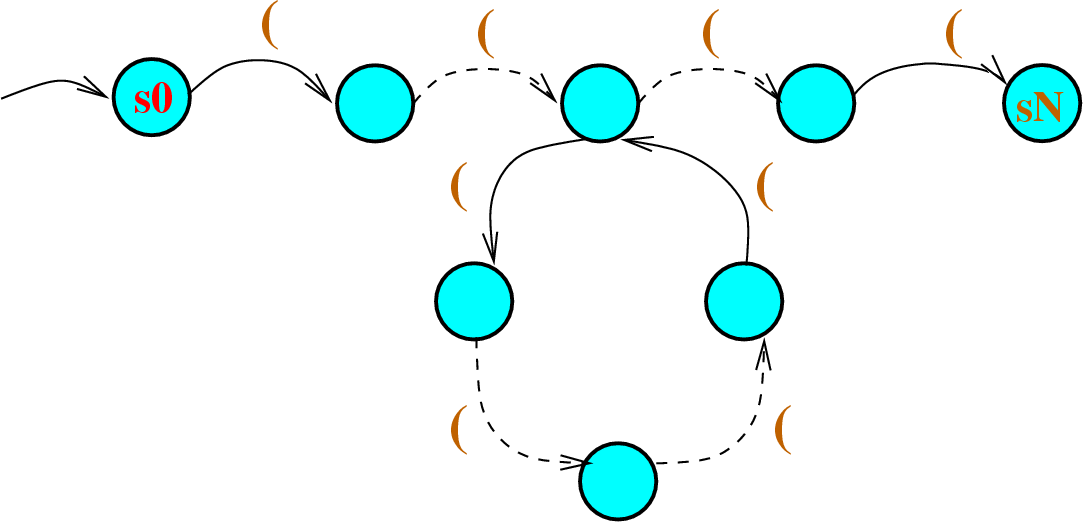Therefore the language of arithmetic expressions overcannot be recognized by FA.

It follows from Remark 1 that finite automata cannot recognize all languages that we may encounter when building a compiler. The notion of a grammar (to be introduced later) solves this difficulty.Next: The notion of a grammar Up: Grammars and Parsing Previous: Grammars and Parsing
Marc Moreno Maza
2004-12-02